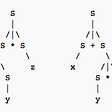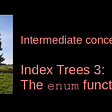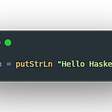# Caesar Cipher: A Simple Encryption System in PicoLisp

## Est omnium rerum magister usus.

Welcome to the “Classic Algorithms” series. Here we will discuss code examples from the Rosetta Code Project and explain step by step how the implementation works. Our first task will be the “Caesar Cipher”.

In this post, we will meet many of the “List and Strings” functions that we know from the Beginner’s-series.

If you want to try by yourself first, here is the task description:

Implement a Caesar cipher, both encoding and decoding. The key is an integer from 1 to 25. This cipher rotates (either towards left or right) the letters of the alphabet (A to Z). The encoding replaces each letter with the 1st to 25th next letter in the alphabet (wrapping Z to A). So key 2 encrypts “HI” to “JK”, but key 20 encrypts “HI” to “BC”.

# How the Caesar Cipher encryption works

If you ever studied cryptographic algorithms, you will probably have come across the Caesar Cipher as it is easy to understand (and easy to break, too).

It is named after the Roman emperor Julius Caesar as there is some evidence that he used this encryption system to transport secret messages. For more historical background, see here.

The idea is that every letter in the alphabet is shifted by a fixed number of steps, which is the “encoding key”. For example, if the key is 2, then you write “C” for “A”, “D” for “B”, “E” for “C” and so on. At the end of the alphabet, “Y” becomes “A” and “Z” becomes “B”. Obviously it is not a very safe encryption because it’s easy to crack using statistical methods, or by checking all possible shifts.

Nevertheless, the implementation of the Caesar Cipher in PicoLisp is a nice example to start our Rosetta code series. It illustrates some interesting concepts such as circular lists, mapping, and anonymous functions. Many of the functions were already introduced in the “PicoLisp for Beginners” series, and the code is elegant and short.

Let’s go!

# An intuitive approach to the algorithm

How should we implement this algorithm? It is clear that the order of the letters is very important, and it needs to be circular since the end of the alphabet should be mapped to its beginning. The picture from the cover sheet illustrates the principle very well:

The inner circle is the plain character, the outer circle is the encryption. Let’s try to implement this in PicoLisp now.

# Step 1 — Creating the alphabet list

The first thing we need is the list of all alphabet characters. One main idea is to make the list “circular”, i. e. after “Z” comes “A” (like the tool on the pic above). In PicoLisp, this can be done by the `.` symbol, for example `(1 2 3 .)` or using the `circ` function. Let's form the list:

The straightforward way: Of course, we can just type each letter by hand.

`(setq *Letters '("A" "B" "C" ... "Z" .))`

The elegent way: The solution above is quite error-prone. A better way would be to use ASCII encoding to create this list. Uppercase letters are encoded by the numbers 65 to 90. So let’s do the following:

1. create a list from 65 to 90 using `(range 65 90)` --> (65 66 67 ... 90).
2. Numbers can be converted to characters by `char`, for example (char 65) = A. Let's apply the `char` function to our list with `(mapcar char (range 65 90))`. --> ("A" "B" "C" ... "Z").
3. Now make the list circular by applying the `circ` function to each letter. `(apply circ (mapcar char(...)))` --> ("A" "B" "C" ... "Z" .), where `.` shows that after "Z" comes "A" again.
4. Set this list to the global variable `*Letters` (with uppercase and asterisk according to naming convention).

We get:

`:(setq *Letters (apply circ (mapcar char (range 65 90))))-> ("A" "B" "C" "D" "E" "F" "G" "H" "I" "J" "K" "L" "M" "N" "O" "P" "Q" "R" "S" "T" "U" "V" "W" "X" "Y" "Z" .)`

# Step 2 — Encoding a single character

Let’s try to get the string we want to encode. As first step, let’s convert all letters to uppercase with `uppc` and use the `chop` function to transform the string into a list of characters:

`:(chop (uppc "in vino veritas")) ->("I" "N" " " "V" "I" "N" "O" " " "V" "E" "R" "I" "T" "A" "S")`

Now to encode these letters, we need to create a function that takes any character and returns the encoded one. Let’s go through it step by step.

1. First we check if the character is within the `*Letters` list by using the `member` function, which returns the list starting from that character if it exists, otherwise `NIL`.
• `: (member "U" *Letters) -> ("U" "V" "W" "X" "Y" "Z" "A" "B" "C" "D" "E" "F" "G" "H" "I" "J" "K" "L" "M" "N" "O" "P" "Q" "R" "S" "T" .) : (member "D" *Letters) -> ("D" "E" "F" "G" "H" "I" "J" "K" "L" "M" "N" "O" "P" "Q" "R" "S" "T" "U" "V" "W" "X" "Y" "Z" "A" "B" "C" .`
• As you can see, the `member` function returns the `*Letters` list starting at the respective letter. Now we want to get the encoded letter, which is shifted in position by the encryption code `key`. In order to shift the list, we use the `nth` function, which takes a list and integer value and returns the tail of the list starting from that value:
• `:(nth (member "U" *Letters) 2)) -> ("V" "W" "X" "Y" "Z" "A" "B" "C" "D" "E" "F" "G" "H" "I" "J" "K" "L" "M" "N" "O" "P" "Q" "R" "S" "T" "U" .) :(nth (member "D" *Letters) 6) -> ("I" "J" "K" "L" "M" "N" "O" "P" "Q" "R" "S" "T" "U" "V" "W" "X" "Y" "Z" "A" "B" "C" "D" "E" "F" "G" "H" .)`
• It’s getting close, but when we check the list returned by `nth`, we actually want the second item in the shifted list, because the `nth` function is only shifting by `(key -1)` positions. To get this second item, we use `cadr`, which is short for `cdr` and `car`. (Re-read this post if you're not sure what `car` and `cdr` mean).
• `: (cadr (nth (member "U" *Letters) 2))) -> "W" : (cadr (nth (member "D" *Letters) 6)) -> "J"`

# Step 3 — Bringing it together

In Step 1 we chopped up the input string to a list, and in Step 2 we managed to encode each of these letters separately. Now let’s bring it together.

We want to take each letter of the input string and apply our encoding function on each single element, which is a typical application for `mapcar`. `mapcar` takes a function and a list as arguments. But what is our function? Obviously, we could define it like this:

`(de encodeChar (C)    (cadr (nth (member C *Letters) Key))) )`

and hand it over to `mapcar`. But actually we need the encoding function only once, so it might be a better option (in terms of structure and readability) to define it as anonymous function.

As we might remember from the beginner’s tutorial, the syntax for anonymous functions is “ ‘((args) ( function )) “, so we can define it as:

`:'((C) (cadr (nth (member C *Letters) Key)))`

where `C` is the character to be encoded.

Now let’s create and test our `mapcar` anonymous function call on the chopped list, with `key = 3`:

`: (setq TestStr (chop (uppc "in vino veritas")))-> ("I" "N" " " "V" "I" "N" "O" " " "V" "E" "R" "I" "T" "A" "S"): (mapcar '((C) (cadr (nth (member C *Letters) 3))) TestStr)-> ("L" "Q" NIL "Y" "L" "Q" "R" NIL "Y" "H" "U" "L" "W" "D" "V")`

This already looks quite promising: at least the character shifting looks correct now.

Now as last step, we need to convert these single letters back to a string, which we can do by using the `pack` function. We wrap it around our `mapcar` function and get:

`: (pack (mapcar '((C) (cadr (nth (member C *Letters) 3))) TestStr))-> "WKLVLVDWHVW"`

As you can see, we “automatically” also got rid of all the `NIL` values that were created by whitespaces and punctuations.

# Step 4 — Finishing the script

Let’s now wrap it up in a small script. We want to call it using the following syntax: `./caesar-cipher.l <plain-string> <key>`, for example:

`\$ ./caesar-cipher.l "In vino veritas" 7PUCPUVCLYPAHZ`

As we learned in the “A very first PicoLisp Program”-post, we can retrieve the two parameters `<plain-string>` and `<key>` using `opt`.

Note that `opt` converts to string, so we need to use the function `format` to convert the key from string to integer, and store both in the global variables `*PlainStr` and `*Key`:

`#! /usr/bin/picolisp /usr/lib/picolisp/lib.l# first command line parameter: plain string(setq *PlainStr (opt))# second command line parameter: key (integer)(setq *Key (format (opt)))`

Also we need to define the global `*Letters` list and f course our encoding function `caesar` that takes a string and a key as parameters and returns the encoded string.

`(setq *Letters (apply circ (mapcar char (range 65 90))))(de caesar (Str Key)   (pack      (mapcar '((C) (cadr (nth (member C *Letters) Key)))         (chop (uppc Str)) ) ) )`

Then finally, we call the `caesar` function with the command line parameters and print out the result.

`(prinl (caesar *PlainStr *Key))`

After that we exit the interpreter with `bye`.

Finished!

`\$ curl https://gitlab.com/picolisp-blog/single-plage-scripts/-/raw/main/rosetta/caesar-cipher.l -o caesar-cipher.l`

Make it executable:

`\$ chmod +x caesar-cipher.l`

Run!

`\$ ./caesar-cipher.l "In vino veritas" 7PUCPUVCLYPAHZ`

This was the first part of the “Rosetta code” series, I hope you liked it.

Tomorrow we will have a look a closer look at the `set` function in PicoLisp, as preparation for the "100 Doors Task" from the Rosetta Code.

# Sources

--

--

--

## More from Mia Temma

These are cross-posts from my blog https://picolisp-explored.com. I’m writing about PicoLisp for beginners. Welcome!

Love podcasts or audiobooks? Learn on the go with our new app.

## Build and Deploy Spring Boot Web Service using Azure DevOps — Part 2## Creating External DSLs using ANTLR and C### The Business Analytics Industry Is A Big Dumpster Fire## Jumanji! Adopting GameDays at Manifold## Ravendex Pre-Sale Details## Enter animation using RecyclerView and LayoutAnimation Part 3: Exclude items## An Intro to Threading in Python## Mia Temma

These are cross-posts from my blog https://picolisp-explored.com. I’m writing about PicoLisp for beginners. Welcome!

## PicoLisp Explored: The enum function## Haskell : Learn the beginner’s way## My first 77 hours with Haskell## Haskell is great but is hard at the same time…